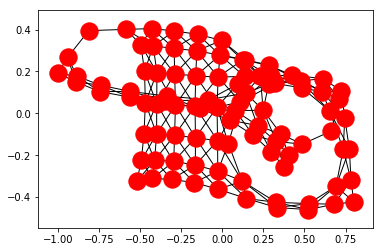# Pathfinding

 Pay Notebook Creator: Salah Ahmed 0 Set Container: Numerical CPU with TINY Memory for 10 Minutes 0 Total 0

# Pathfinding w/ obstacles¶

In :
# CrossCompute
obstacle_table_path = 'obstacles.csv'
source_x = 0
source_y = 0
target_x = 9
target_y = 9
target_folder = '/tmp'

In :
%pylab inline

Populating the interactive namespace from numpy and matplotlib

In :
try:
import cairosvg
except ImportError:
import pip
pip.main(['install', 'cairosvg'])
import cairosvg

Collecting cairosvg
Collecting cairocffi (from cairosvg)
Collecting defusedxml (from cairosvg)
Collecting cssselect2 (from cairosvg)
Requirement already satisfied: pillow in /usr/lib64/python3.6/site-packages (from cairosvg)
Collecting tinycss2 (from cairosvg)
Requirement already satisfied: cffi>=1.1.0 in /usr/lib64/python3.6/site-packages (from cairocffi->cairosvg)
Requirement already satisfied: olefile in /usr/lib/python3.6/site-packages (from pillow->cairosvg)
Requirement already satisfied: webencodings>=0.4 in /home/user/.virtualenvs/crosscompute/lib/python3.6/site-packages (from tinycss2->cairosvg)
Requirement already satisfied: pycparser in /usr/lib/python3.6/site-packages (from cffi>=1.1.0->cairocffi->cairosvg)
Building wheels for collected packages: cairocffi
Running setup.py bdist_wheel for cairocffi: started
Running setup.py bdist_wheel for cairocffi: finished with status 'done'
Stored in directory: /home/user/.cache/pip/wheels/ed/67/1b/c2e45826aef8e76b0942c2c1e62120da72259a5c1e46a3d02d
Successfully built cairocffi
Installing collected packages: cairocffi, defusedxml, tinycss2, cssselect2, cairosvg
Successfully installed cairocffi-0.8.0 cairosvg-2.1.3 cssselect2-0.2.1 defusedxml-0.5.0 tinycss2-0.6.1

In :
import itertools
import math
import networkx as nx

from os.path import join
from shapely import wkt
from shapely.geometry import MultiPolygon, GeometryCollection, Point, LineString

source_xy = int(source_x), int(source_y)
target_xy = int(target_x), int(target_y)
obstacle_polygons = [wkt.loads(x) for x in obstacle_table['WKT']]
mp = MultiPolygon(obstacle_polygons)
mp

Out:
<shapely.geometry.multipolygon.MultiPolygon at 0x7f2e94712f60>
In :
target_path = join(target_folder, 'obstacles.png')
cairosvg.svg2png(bytestring=mp._repr_svg_(), write_to=target_path)
print('obstacles_image_path = %s' % target_path)

obstacles_image_path = /tmp/obstacles.png

In :
def get_points(pointa, pointb, obstacles):
''' returns all nodes for coordinate grid
params:
@pointa: starting point, (int, int)
@pointb: end point, (int, int)
@obstacles: group of polygons, shapely.geometry.MultiPolygon
'''
bounds = list(itertools.chain(pointa, pointb, obstacles.bounds))
minimum, maximum = int(min(bounds)), int(max(bounds))
coords = itertools.product(range(minimum, maximum + 1), range(minimum, maximum + 1))
points = []
for i in coords:
point = Point(*i)
if all([(
not obstacle.contains(point)) for obstacle in obstacles.geoms]):
points.append(point)
return points

In :
points = get_points(source_xy, target_xy, mp)

In :
G = GeometryCollection(obstacle_polygons + points)
G

Out:
<shapely.geometry.collection.GeometryCollection at 0x7f2e56c8a048>
In :
target_path = join(target_folder, 'grid.png')
cairosvg.svg2png(bytestring=G._repr_svg_(), write_to=target_path)
print('grid_image_path = %s' % target_path)

grid_image_path = /tmp/grid.png

In :
nodes = [(c.coords.xy, c.coords.xy) for c in points]
nodes[:10]

Out:
[(0.0, 0.0),
(0.0, 1.0),
(0.0, 2.0),
(0.0, 3.0),
(0.0, 4.0),
(0.0, 5.0),
(0.0, 6.0),
(0.0, 7.0),
(0.0, 8.0),
(0.0, 9.0)]
In :
graph = nx.Graph()
for n in nodes:
adj_nodes = [(n + 1, n), (n, n + 1), (n - 1, n), (n, n - 1)]
dnodes = [(n + 1, n + 1),
(n - 1, n + 1), (n - 1, n - 1), (n + 1, n - 1)]
d_nodes = filter(
lambda c: c in nodes and all([
not obstacle.contains(
LineString([n, c])
) for obstacle in obstacle_polygons
]), dnodes)
for i in d_nodes:

In :
nx.draw_networkx(graph, with_labels=False)from shapely.geometry import LineString
line = LineString([
(0, 0),
(5, 0),
(5, 5),
(3, 5),
(3, 9),
(9, 9),
])
line

In :
# !!! Put a pathfinding algorithm here
path = nx.algorithms.dijkstra_path(graph, source_xy, target_xy)
line = LineString(path)
line

Out:
<shapely.geometry.linestring.LineString at 0x7f2e5232f908>
In :
target_path = join(target_folder, 'line.png')
cairosvg.svg2png(bytestring=line._repr_svg_(), write_to=target_path)
print('line_image_path = %s' % target_path)

line_image_path = /tmp/line.png

In :
gc = GeometryCollection(obstacle_polygons + [
Point(source_xy),
Point(target_xy),
line,
])

In :
target_path = join(target_folder, 'collection.png')
cairosvg.svg2png(bytestring=gc._repr_svg_(), write_to=target_path)
print('collection_image_path = %s' % target_path)

collection_image_path = /tmp/collection.png

In :
from pandas import DataFrame
waypoint_table = DataFrame(path, columns=['x', 'y'])
waypoint_table

Out:
<style scoped> .dataframe tbody tr th:only-of-type { vertical-align: middle; } .dataframe tbody tr th { vertical-align: top; } .dataframe thead th { text-align: right; } </style>
x y
0 0.0 0.0
1 1.0 0.0
2 2.0 0.0
3 3.0 0.0
4 4.0 1.0
5 5.0 2.0
6 6.0 3.0
7 7.0 4.0
8 8.0 5.0
9 9.0 6.0
10 9.0 7.0
11 9.0 8.0
12 9.0 9.0
In :
waypoint_table_path = join(target_folder, 'waypoints.csv')
waypoint_table.to_csv(waypoint_table_path, index=False)

In :
print('optimal_path_table_path = %s' % waypoint_table_path)

optimal_path_table_path = /tmp/waypoints.csv